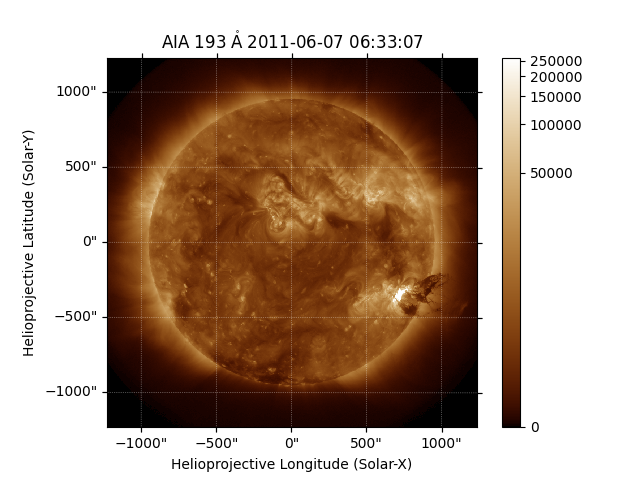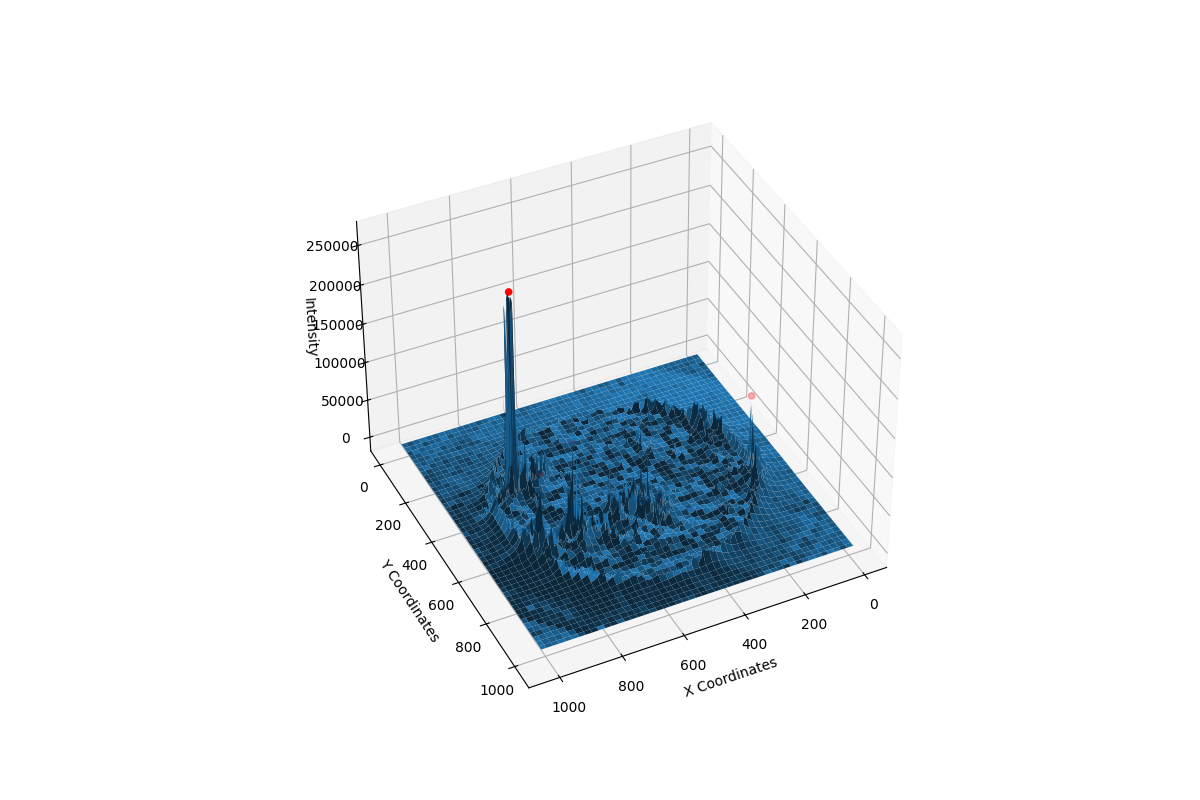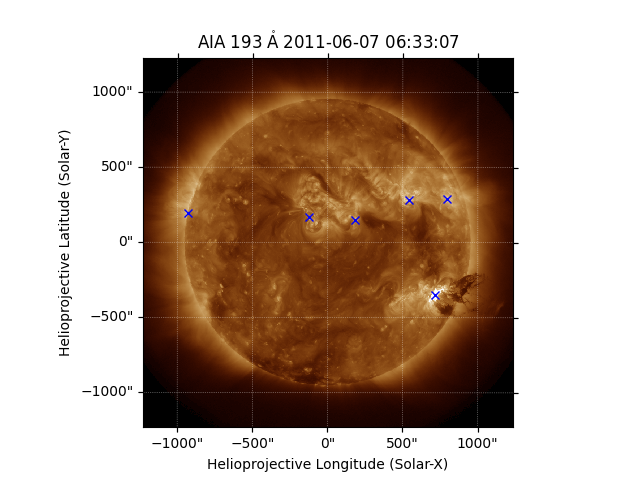# Finding Local Peaks in Solar Data#

Detecting intensity peaks in solar images can be useful, for example as a simple flare identification mechanism. This example illustrates detection of areas where there is a spike in solar intensity. We use the peak_local_max function in the scikit-image library to find those regions in the map data where the intensity values form a local maxima. Then we plot those peaks in the original AIA plot.

import matplotlib.pyplot as plt
from skimage.feature import peak_local_max

import astropy.units as u

import sunpy.map
from sunpy.data.sample import AIA_193_IMAGE
from sunpy.map.maputils import all_pixel_indices_from_map


We will first create a Map using some sample data and display it.

aiamap = sunpy.map.Map(AIA_193_IMAGE)
fig = plt.figure()
aiamap.plot(axes=ax)
plt.colorbar()<matplotlib.colorbar.Colorbar object at 0x7fb37e35b880>


Before we find regions of local maxima, we need to create some variables to store pixel values for the 2D SDO/AIA data we are using. These variables are used for plotting in 3D later on.

X, Y = all_pixel_indices_from_map(aiamap)


We will only consider peaks within the AIA data that have minimum intensity value equal to threshold_rel * max(Intensity) which is 20% of the maximum intensity. The next step is to calculate the pixel locations of local maxima positions where peaks are separated by at least min_distance = 60 pixels. This function comes from scikit image and the documentation is found here peak_local_max.

coordinates = peak_local_max(aiamap.data, min_distance=60, threshold_rel=0.2)


We now check for the indices at which we get such a local maxima and plot those positions marked red in the aiamap data.

fig = plt.figure(figsize=(12, 8))
ax.plot_surface(X, Y, aiamap.data)
ax.view_init(elev=39, azim=64)
peaks_pos = aiamap.data[coordinates[:, 0], coordinates[:, 1]]
ax.scatter(coordinates[:, 1], coordinates[:, 0], peaks_pos, color='r')
ax.set_xlabel('X Coordinates')
ax.set_ylabel('Y Coordinates')
ax.set_zlabel('Intensity')
plt.show()Now we need to turn the pixel coordinates into the world location so they can be easily overlaid on the Map.

hpc_max = aiamap.wcs.pixel_to_world(coordinates[:, 1]*u.pixel, coordinates[:, 0]*u.pixel)


Finally we do an AIA plot to check for the local maxima locations which will be marked with a blue x-label.

fig = plt.figure()